# Exploratory analysis

Data visualization, part 1. Code for Quiz 7.

1. Load the R packages we will use
``````library(tidyverse)
``````
1. Quiz Questions
• Replace all the ???s. These are answers on your moodle quiz.
• Run all the individual code chunks to make sure the answers in this file correspond with your quiz answers
• After you check all your code chunks run then you can knit it. It won’t knit until the ??? are replaced
• The quiz assumes you have watched the videos had worked through the exercises in exercises_slides-1-49.Rmd
1. Pick one of your plots to save as your preview plot. Use the ggsave command at the end of the chunk of the plot that you want to preview.

# Question: Modify Slide 34

• Create a plot with the `faithful` dataset
• Add points with `geom_point`
• Assign the variable `eruptions` to the x-axis
• Assign the variable `waiting` to the y-axis -color the points according to whether `waiting` is smaller or greater than 81
``````ggplot(faithful) +
geom_point(aes(x = eruptions, y = waiting,
colour = waiting > 81))
``````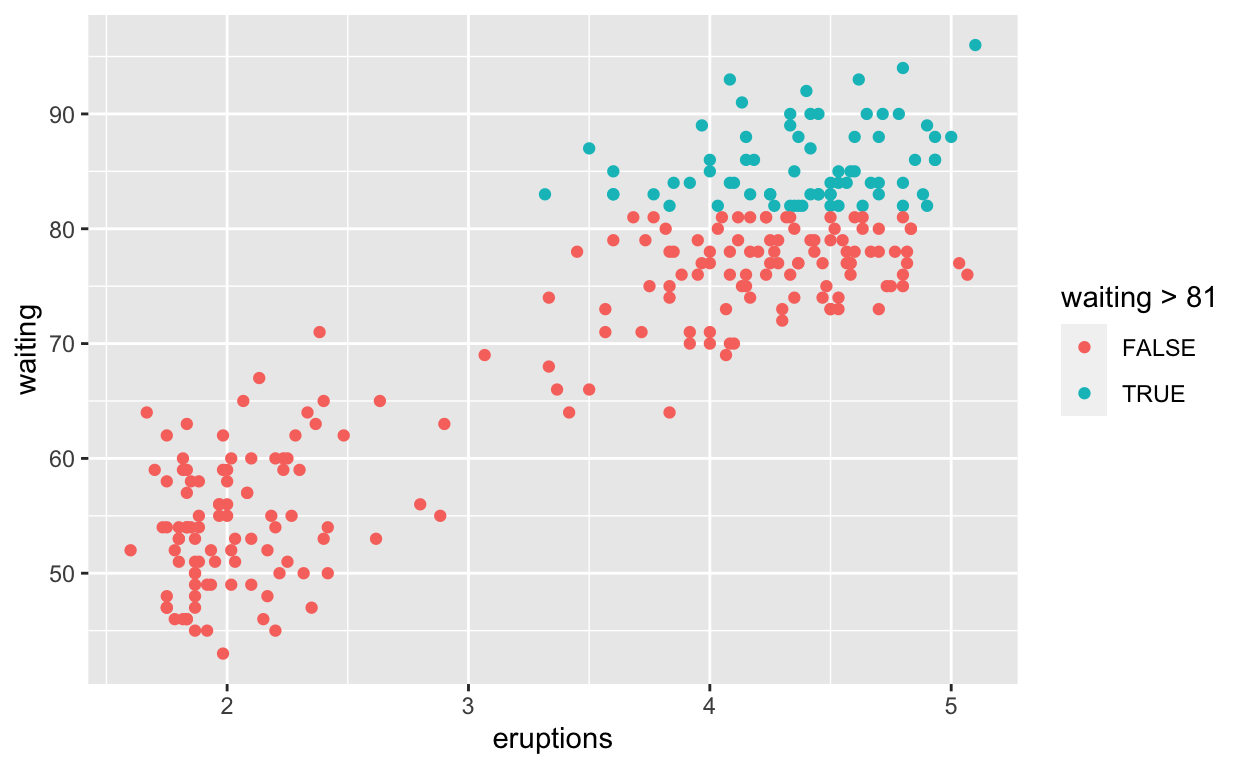# Question: Modify Intro-Slide 35

• Create a plot with the `faithful` dataset
• Add points with `geom_point`
• Assign the variable `eruptions` to the x-axis
• Assign the variable `waiting` to the y-axis
• Assign the color purple to all the points
``````ggplot(faithful) +
geom_point(aes(x = eruptions, y = waiting),
colour = "purple")
``````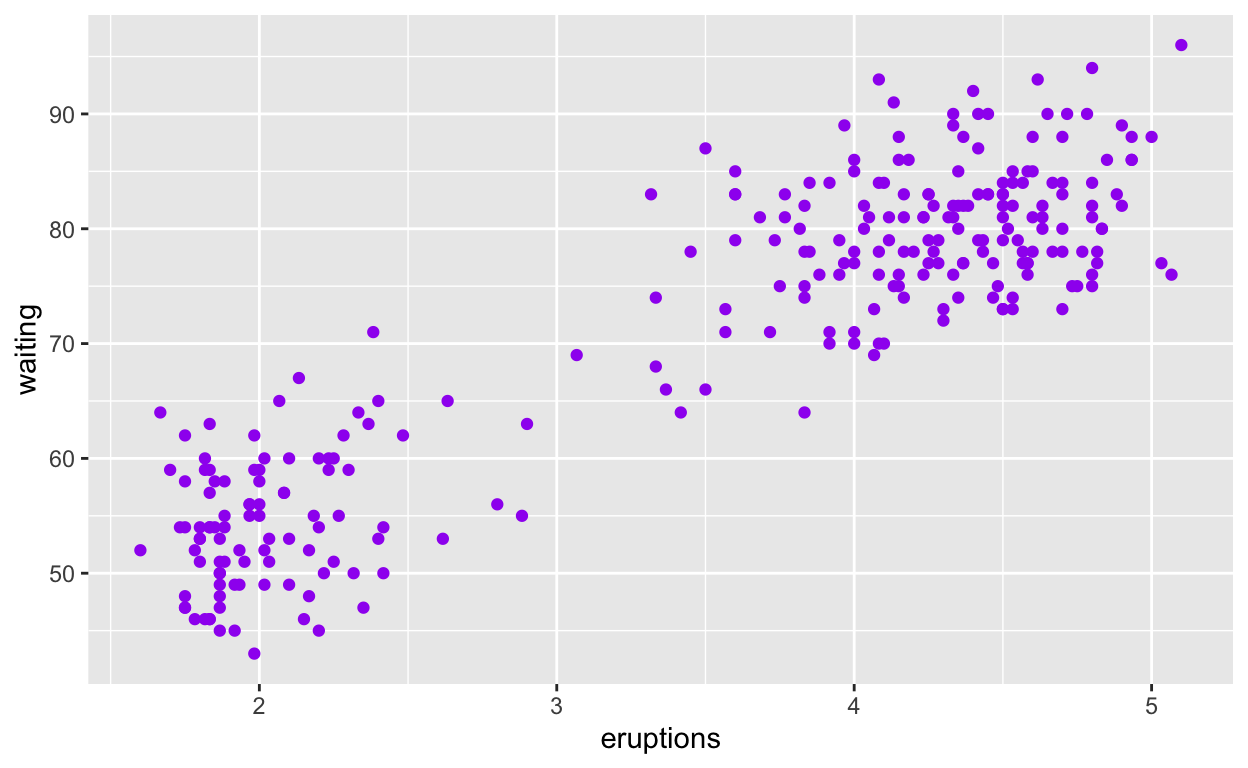# Question: Modify Intro-Slide 36

• Create a plot with the `faithful` dataset
• Use `geom_histogram()` to plot the distribution of `waiting` time
• Assign the variable `waiting` to the x-axis
``````ggplot(faithful) +
geom_histogram(aes(x = waiting))
``````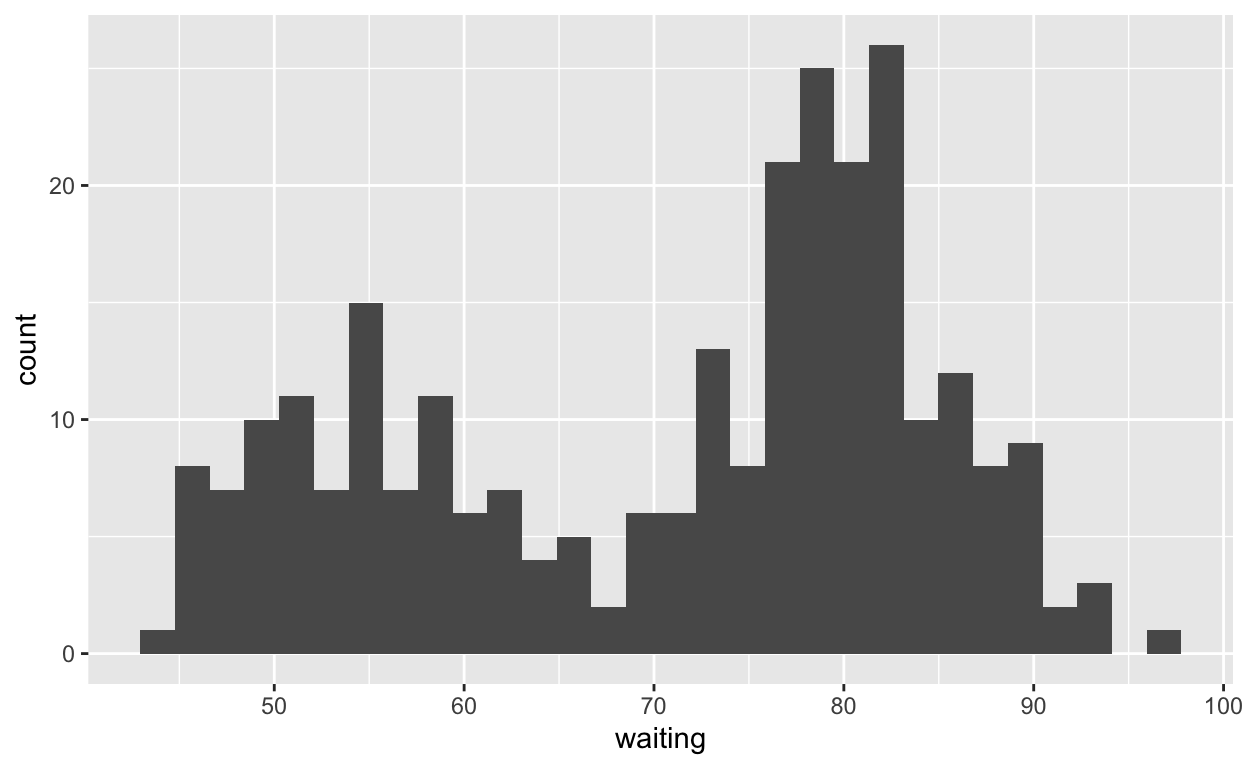# Question: Modify geom-ex-1

• See how shapes and sizes of points can be specified here: https://ggplot2.tidyverse.org/articles/ggplot2-specs.html#sec:shape-spec
• Create a plot with the `faithful` dataset
• Add points with `geom_point`
• Assign the variable `eruptions` to the x-axis
• Assign the variable `waiting` to the y-axis
• Set the shape of the points to plus
• Set the point size to 1
• Set the point transparency 0.4
``````ggplot(faithful) +
geom_point(aes(x = eruptions, y = waiting),
shape = "plus", size = 1, alpha =0.4)
``````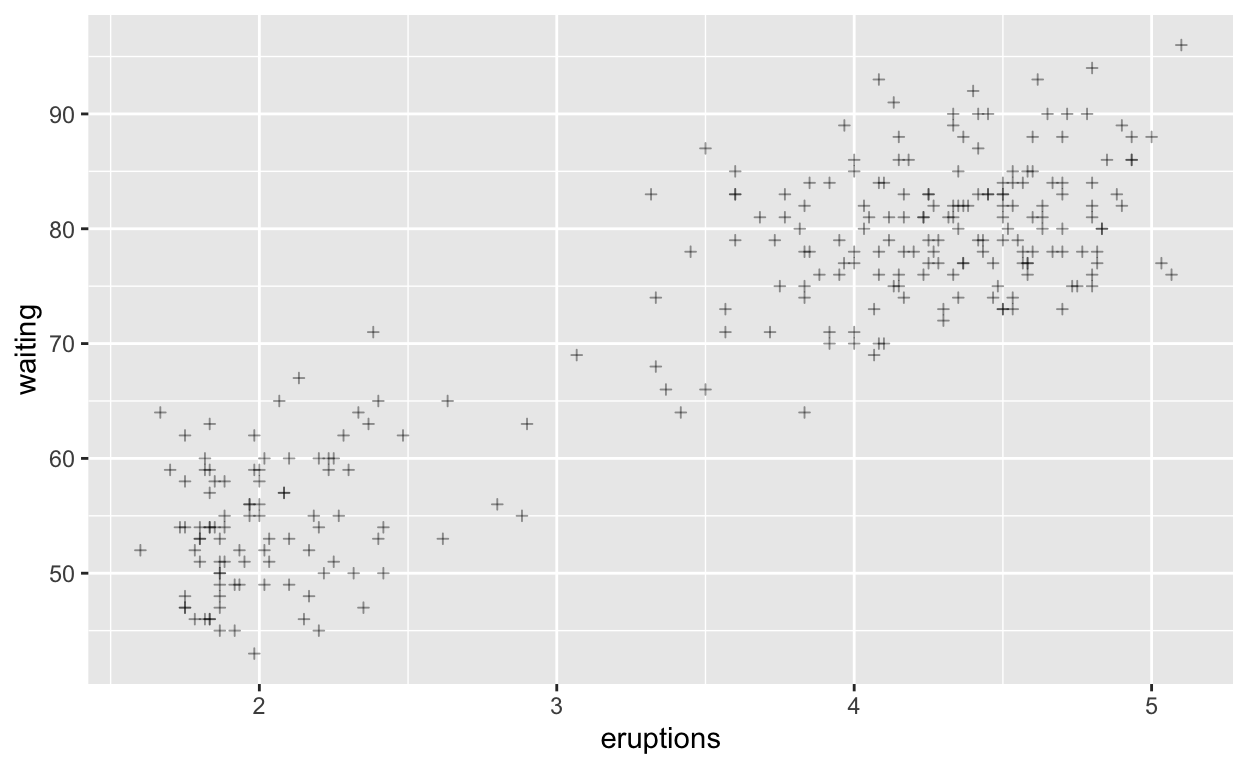# Question: Modify geom-ex-2

• Create a plot with the `faithful` dataset
• Use `geom_histogram()` to plot the distribution of the `eruptions` (time)
• Fill in the histogram based on whether `eruptions` are greater than or less than 3.2 minutes
``````ggplot(faithful) +
geom_histogram(aes(x = eruptions, fill = eruptions > 3.2 ))
``````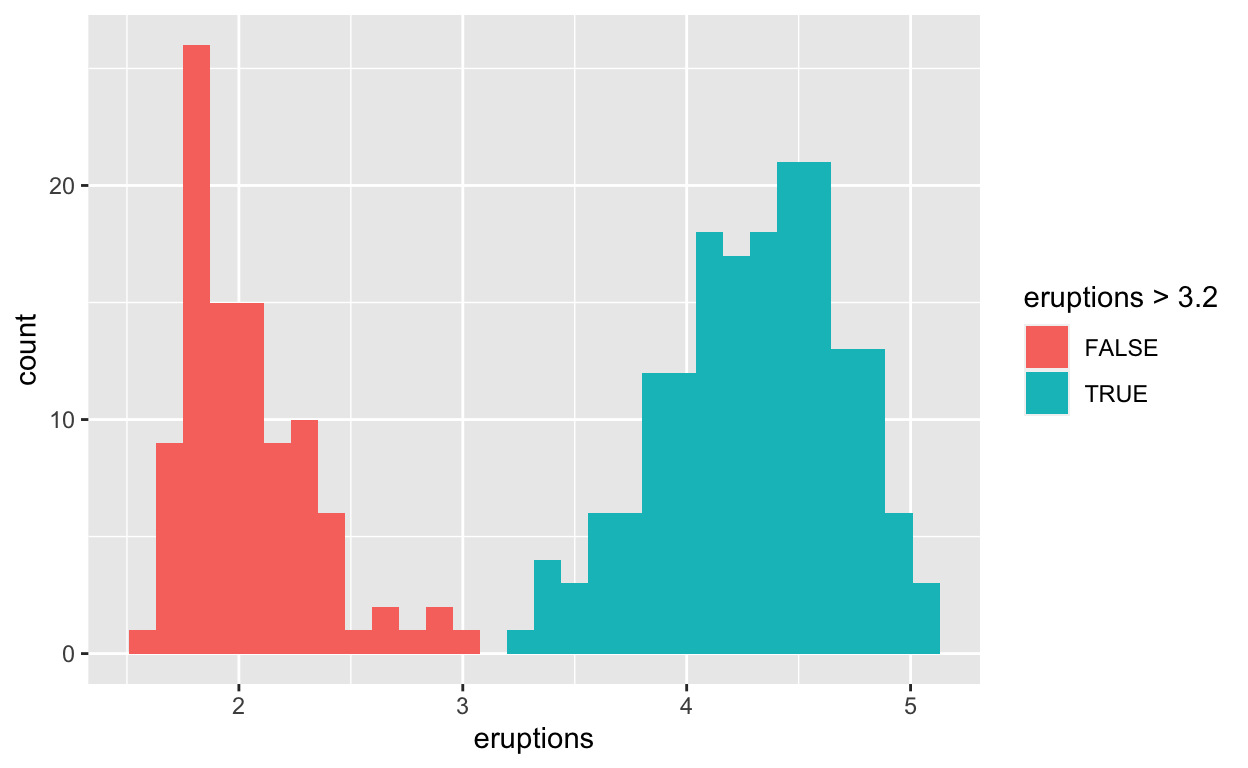# Question: Modify stat-slide-40

• Create a plot with the `mpg` dataset
• Add `geom_bar()` to create a bar chart of the variable `manufacturer`
``````ggplot(mpg) +
geom_bar(aes(x = manufacturer))
``````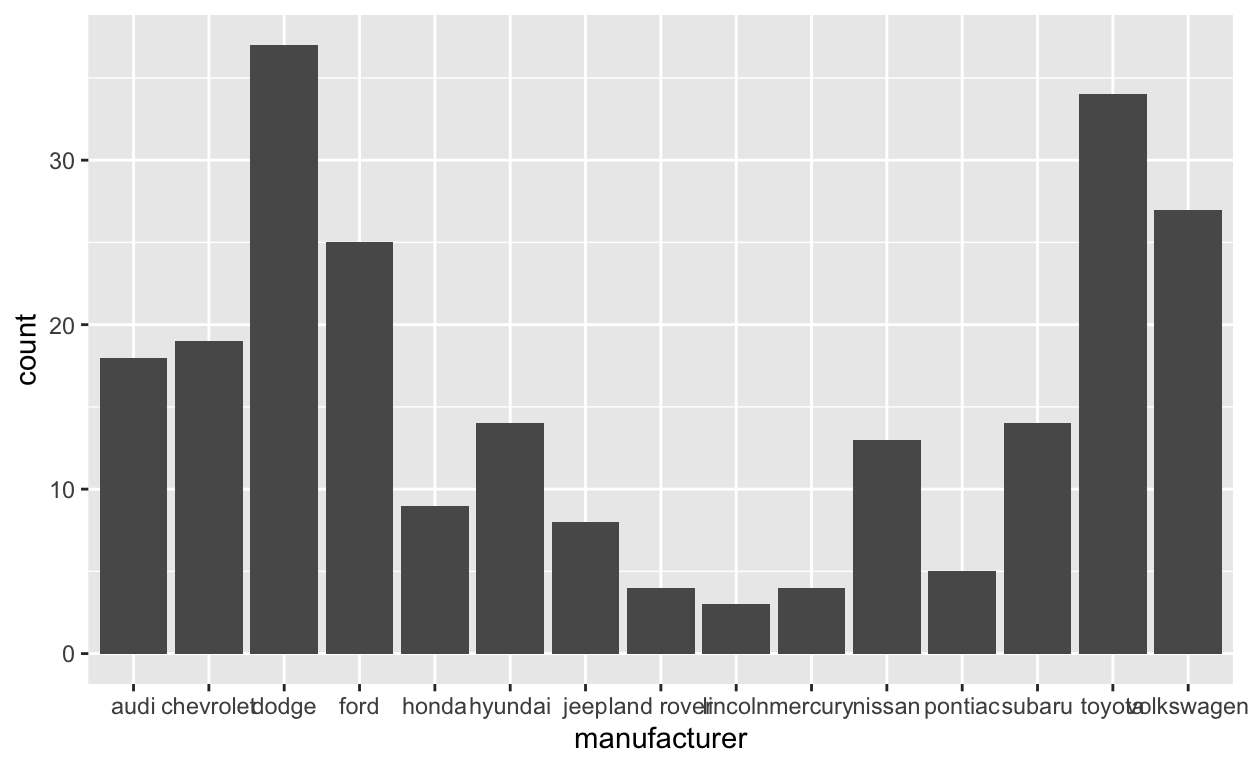# Question: Modify stat-slide-40

• Change code to count and to plot the variable `manufacturer` instead of `class`
``````mpg_counted <- mpg %>%
count(manufacturer, name = 'count')
ggplot(mpg_counted) +
geom_bar(aes(x = manufacturer, y = count), stat = 'identity')
``````# Question: Modify stat-slide-43

• Change code to plot bar chart of each `manufacturer` as a percent of total
• Change `class` to `manufacturer`
``````ggplot(mpg) +
geom_bar(aes(x = manufacturer, y = after_stat(100 * count / sum(count))))
``````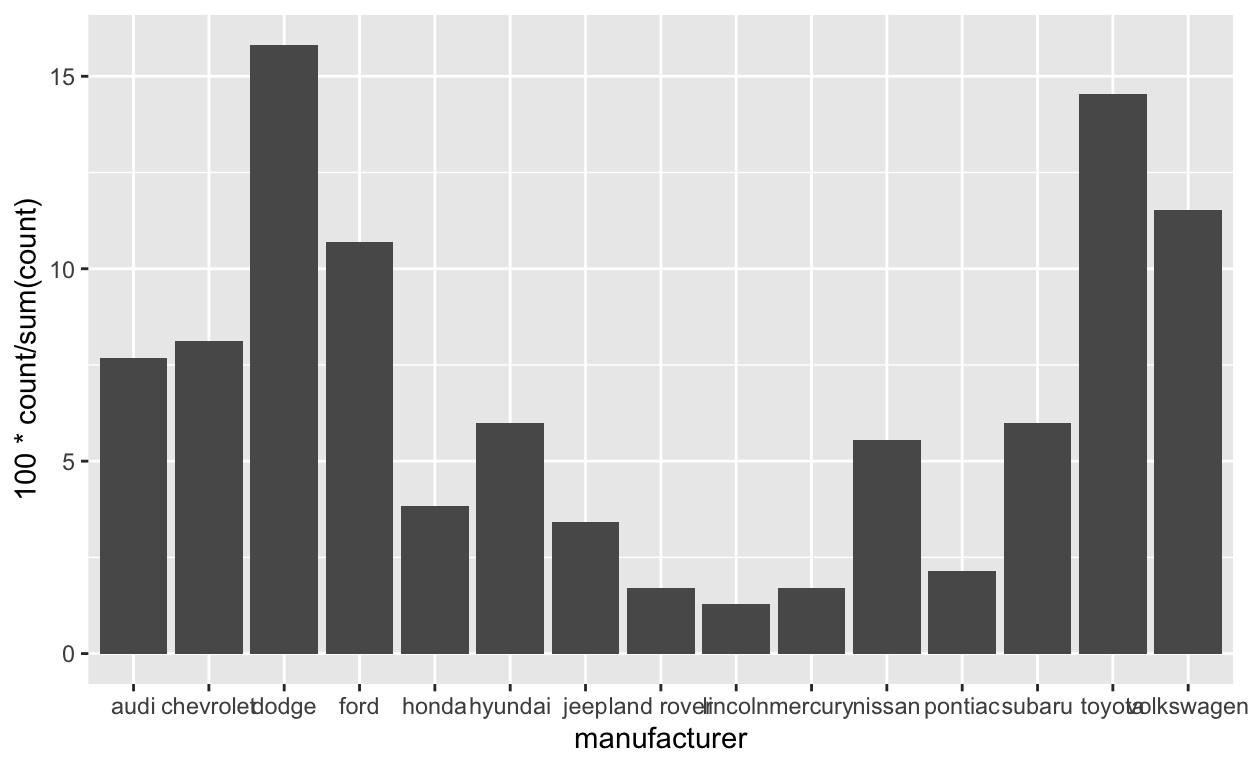# Question: Modify answer to stat-ex-2

``````ggplot(mpg) +
geom_jitter(aes(x = class, y = hwy), width = 0.2) +
stat_summary(aes(x = class, y = hwy), geom = "point",
fun = "median", color = "purple3",
shape = "square", size = 8 )
``````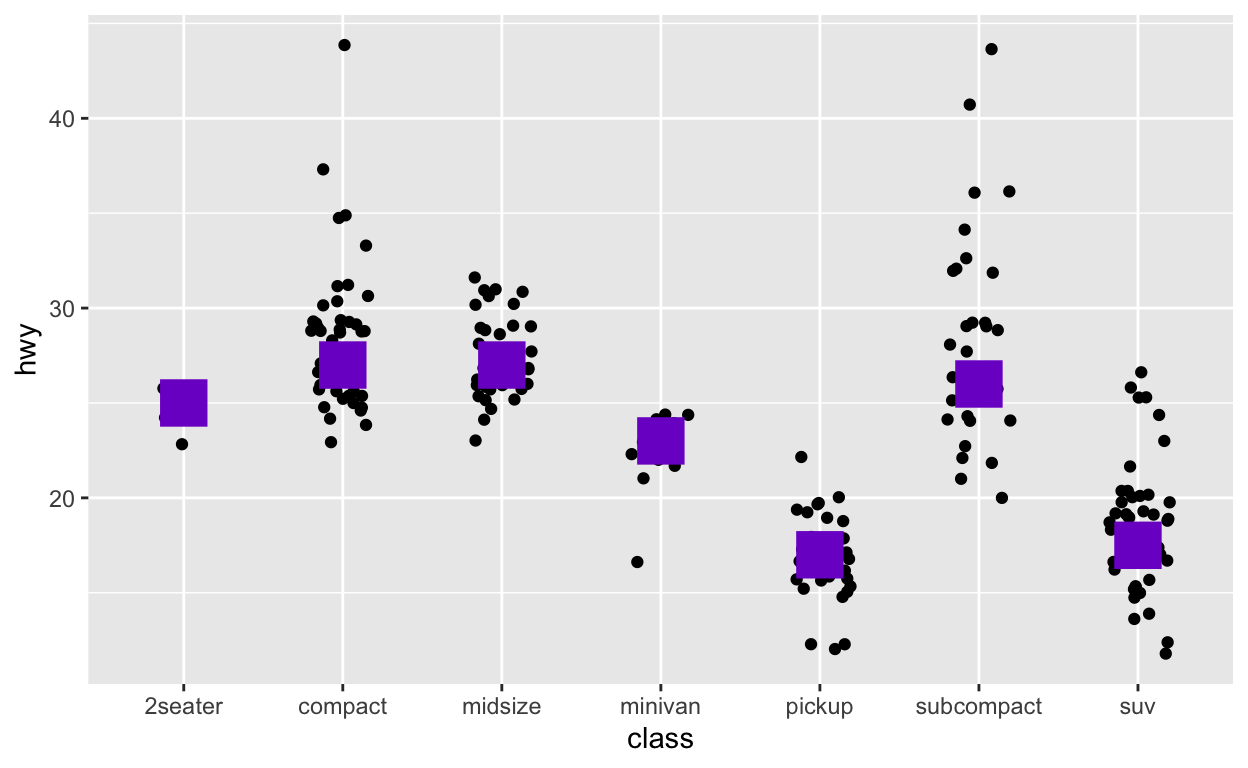``````ggsave(filename = "preview.png",
path = here::here("_posts", "2022-03-17-exploratory-analysis"))
``````